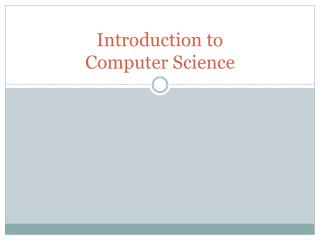DownloadDownload PresentationIntroduction to Computer Science

# Introduction to Computer Science

Download Presentation## Introduction to Computer Science

- - - - - - - - - - - - - - - - - - - - - - - - - - - E N D - - - - - - - - - - - - - - - - - - - - - - - - - - -
##### Presentation Transcript

1. Introduction to Computer Science

2. A Quick Puzzle • Well-Formed Formula • any formula that is structurally correct • may be meaningless • Axiom • A statement that is defined to be true • Production Rule • A rule that generates a true statement from another true statement • Valid Statement • A statement that is true

3. P-Q System • The P-Q Language • A statement in P-Q is WFF if and only if it is made up of the symbols “-,p,q”, and has 0 or more dashes, followed by p, followed by 0 or more dashes, followed by q, followed by 0 or more dashes • --------p------q---- is WFF • ---q----p--- is not WFF http://en.wikipedia.org/wiki/G%C3%B6del,_Escher,_Bach

4. P-Q Production Rules • Production rule: • if xpyqzis a valid statement, then so is xpy – qz – • Example: • if - - - - - p - - z - - - is a valid statement, then so is - - - - - p - - - z - - - - • Can we derive an algorithm to decide if a given statement in P-Q is well formed? • Can we derive an algorithm to decide if a given statement is is valid?

5. P-Q Meaning • What if we let p be plus, and q be equal? • Suppose we start with the statement pq • Is it WFF? Is it valid? • Suppose we apply the production rule once? • pq => p-q- • Suppose we start with –pq? • -pq = -p-q—

6. P-Q is Addition • P-Q is really isomorphic with addition • There is a clear equivalence between a statement in p-q, and a statement involving integer addition. • Can we prove that a given statement is valid? • Sure – start with the 0 statement • Keep applying the production rule until you either find the string (true) or until you find strings that are longer (false) • Can we list all statements in P-Q?

7. MIU Language • The MIU Language • A statement in MIU is WFF if an only if it contains the characters M,I,and U, and M is the first character • Production Rules • Given a string Mx => Mxx • Given a string ends in I, replace it with U • Given a III in a string, replace it with U • Given UU in a string, remove them • Examples: • MUIUI => MUIUU => MUI => MUU => M • MIIII => MUI => MUU => M • Given the string MI, can we arrive at MU? http://en.wikipedia.org/wiki/G%C3%B6del,_Escher,_Bach

8. Wait, what? • P-Q • Had a nice decision procedure • It was straight-forward to derive new facts, and we knew when to stop trying • MIU • Has NO nice decision procedure • It also has a straight-forward way to derive new facts, but we didn’t know when to stop • Difference? • What does this MEAN for us?

9. Gödel's first incompleteness theorem, states that: • Any effectively generated theory capable of expressing elementary arithmetic cannot be both consistent and complete. In particular, for any consistent, effectively generated formal theory that proves certain basic arithmetic truths, there is an arithmetical statement that is true, but not provable in the theory. • MIU is an example of this • We cannot derive MU from MI http://en.wikipedia.org/wiki/G%C3%B6del%27s_incompleteness_theorems

10. But Godel was a Philosopher… • Entscheidungsproblem • Proved by Church & Turing in 1936 – • The Entscheidungsproblem asks for an algorithm that will take as input a description of a formal language and a mathematical statement in the language and produce as output either "True" or "False" according to whether the statement is true or false. The algorithm need not justify its answer, nor provide a proof, so long as it is always correct. Such an algorithm would be able to decide, for example, whether statements such as Goldbach's conjecture or the Riemann hypothesis are true, even though no proof or disproof of these statements is known. • Church & Turing (and us) demonstrated that it is not possible for any such system to exist for all such formal languages.

11. So what? • We just proved that • There are formal languages that we cannot write decision procedures to verify validity of a statement • Computer programs are formal languages, and we cannot write a program to determine when a computer program is valid • Even worse still, we cannot write a computer program to tell when we are running in a system like P-Q or like MIU – we just have to wait for the answer – possibly forever

12. Wait, WHAT? • Computer Science • Before the first computers we know • that there are problems we can solve • that there are problems we cannot solve • that there are problems that if we can solve them at all are going to take a very very long time • and that these things are true no matter how fast our systems get • We now know that • We can tell the difference between “hard” and “easy” problems • We can often write approximations to the real systems • And when we can’t, we need to know this before we waste our time trying